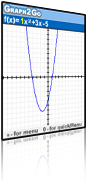Graph2Go 0.84Graph2Go is a special-purpose graphing calculator that operates for given sets of function expressions.

### Description

Graphing calculators are instrumental in teaching and learning mathematics. It is an environment that supports conceptual understanding of functions in general, and school algebra and real analysis in particular. Especially, it enhances connections between graphic and symbolic representations. A major objective of algebra teaching is equipping learners with tools to mathematize their perceptions. A multi-representational approach has the potential to shift the focus of solving even traditional problems from assigning and solving for an unknown to analyzing the various processes and relations among those processes. The integration of multiple representations of function creates opportunities for developing a wider range of solution methods to traditional problems. Zooming in on the use of the graphing calculator, researchers point on four patterns and modes of use: computational tool, data analysis tool, visualizing tool, and checking tool.

Dynamic transformations are a unique facility of Graph2Go.

Dynamic control involves the direct manipulation of an object or a representation of a mathematical object. As the driving input is the letter-symbolic one, the transformations are carried out on the numbers involved in the function’s expression. Thus, by parameterizing an example we turn it into a family of functions. Research suggests that the kinesthetic relation between the user and the object on the screen can have an important role in developing a deeper understanding of the mathematical concept.

### Features

Basic features of Graph2Go:

• Graphs of single variable function expressions.
• Dynamic graphing of transformed expressions.
• Points of interest (maximum, minimum, inflection_ are marked and their numerical values are presented.
• Graph and expression of the derivative function.
• Graph, expression of the integral function’s family.
• Area expressed by the integral of a given function.
• Zooming and rescaling options.

Graph2Go is a special purpose graphing calculator that operates for given sets of function expressions. Additional sets will become available for downloading from this site. The given families of function expressions and the tools that support easy changes of any given example have been designed for fast and easy use with the small keyboard.

### Suggested Activities

Below is an interesting example that combines the use of visual thinking with an analytic task and has the potential to enhance procedural operations with conceptual understanding:

Prove or refute each of the following statements.

Explain and demonstrate your method and answer using Graph2Go, paper and pencil, or mental operations

• The derivative of a family of functions cannot be a single function.
• [k*f(x)]‘ is equal to k*[f(x)]‘
• [k*(f(x)]‘ is equal to [f(kx)]‘

If you study calculus with the support of graphing calculators, see the AP (Advanced Placement) calculus site for examples of calculus assessment problems (carried out with or without graphing tools).